• ## 资源分配问题

千次阅读 多人点赞 2020-07-20 15:14:18
某工业生产部门根据国家计划的安排，拟将某种高效率的5台机器，分配给所属的3个工厂A，B，C， 各工厂在获得这种机器后，可以为国家盈利的情况如表1所示。问：这5台机器如何分配给各工厂，才能使得国家利益最大？ ...
资源分配问题

大家好，我叫亓官劼（qí guān jié ），在CSDN中记录学习的点滴历程，时光荏苒，未来可期，加油~博客地址为：亓官劼的博客

本文原创为亓官劼，请大家支持原创，部分平台一直在盗取博主的文章！！！

博主目前仅在CSDN中写博客，唯一博客更新的地址为：亓官劼的博客题目
某工业生产部门根据国家计划的安排，拟将某种高效率的5台机器，分配给所属的3个工厂A，B，C， 各工厂在获得这种机器后，可以为国家盈利的情况如表1所示。问：这5台机器如何分配给各工厂，才能使得国家利益最大？
利益表：

<

S
A
B
C


展开全文• 用遗传算法解决资源分配问题，翟旭，，资源分配问题是一种特殊的装箱问题，其实质是在生产资料数量既定的条件下，如何选择生产部门，确定生产规模，寻求产品与产品之间
• 上一篇文章写了动态规划求解0-1背包问题，这里做一道资源分配问题强化理解，顺便分析一下动态规划算法的优化问题。 问题描述：某厂根据计划安排，拟将n台相同的设备分配给m个车间，各车间获得这种设备后，可以为...
上一篇文章写了动态规划求解0-1背包问题，这里做一道资源分配问题强化理解，顺便分析一下动态规划算法的优化问题。
问题描述：某厂根据计划安排，拟将n台相同的设备分配给m个车间，各车间获得这种设备后，可以为国家提供盈利Ci j(i台设备提供给j号车间将得到的利润，1≤i≤n，1≤j≤m) 。问如何分配，才使国家得到最大的盈利？
分析递推公式： 前 i 台设备分配给前 j 个车间，考虑当前第 j 个车间的情况，假设第 j 个车间分配了 k(0 <= k <= i) 个设备，剩下的 i - k 台设备要分给前 j - 1 个车间，此时前 j 个车间利润为p[i][j] = p[i - k][j - 1] + c[k][j]，遍历k的情况可取到最大利润，故有下面公式其中0<=k<=i，1<=i<=n,1<=j<=m
代码如下：
int i, j, k;
for (i = 1; i <= n; i++)
for (j = 1; j <= m; j++)
for (k = 0; k <= i; k++)//i代表了设备数目
if ((p[k][j - 1] + c[i - k][j]) > p[i][j])
{
p[i][j] = p[k][j - 1] + c[i - k][j];
f[i][j] = i - k;//用于当前计算的车间的设备数
}

JS代码
const c = [[0,0,0],[0,189, 152],[0,200, 333]]
function dynamic (c) {
let n = c.length - 1
let m = c.length - 1
let p = (new Array(n + 1).fill(null)).map(() => new Array(m + 1).fill(0))
for (let i = 1; i <= n; i++) {
for (let j = 1; j <= m; j++) {
for (let k = 1; k <= i; k++) { // i代表了设备数目
// 给j号车间提供多少个设备可以使得利润最大化
if (p[i - k][j - 1] + c[k][j] > p[i][j]) {
p[i][j] = p[i - k][j - 1] + c[k][j]
}
}
}
}
return p[n][m]
}
console.log(dynamic(c))

优化一： 如果你在一个实例中尝试一下自己画表，你会发现，这道公式是按列计算数值的。 举个例子，2台设备分配给2个车间的盈利表（c[i][j]）如图所示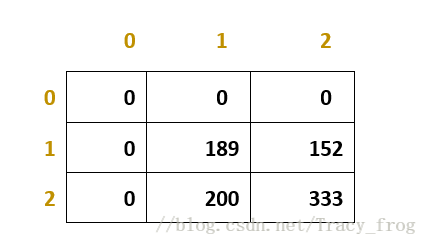初始化最大盈利表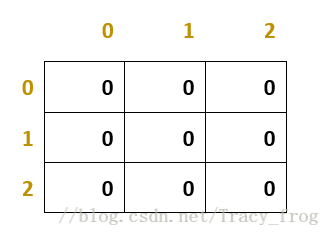根据公式填表(p[i][j]=max{p[i][j],p[k][j-1]+c[i-k][j]} ) p=max{p,p[k]+c[1-k]},0<=k<=1 p=max{p,p+c,p+c}=max{0,189,0}=189 用于计算的有下面4个数字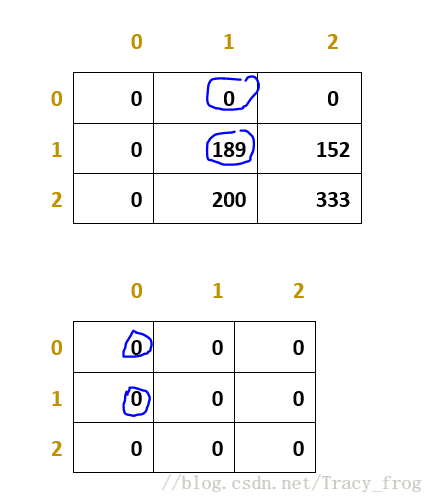这样看或许还不够明确，我们来算一下p p=max{p,p[k]+c[2-k]},0<=k<=2 p=max{p,p+c,p+c,p+c} 用于计算的有下面几个数字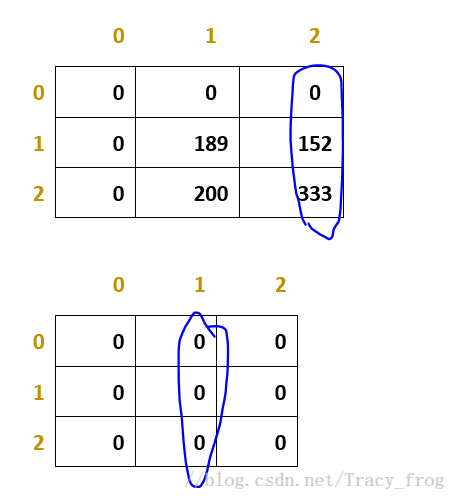问题浮出水面==>这条递推公式是按照列遍历的！！学过计算机组成原理的朋友可能已经知道了，没错，就是cache的问题。 改变一下存储方式即可让遍历按照行进行！
p[i][j]=max{p[i][j],p[i-1][k]+c[i][j-k]} 其中0<=k<=j，1<=i<=m,1<=j<=n 解释一下这条新公式：其实思想没改变，就是将i、j互换，这里i代表车间数，j代表设备数，公式表示前i个车间瓜分j台设备，考虑当前第i个车间的情况，当前车间可能分配0个设备、1个设备、2个设备、…、n个设备，这其中得到最大利润的方案即为最优方案。 注意c[i][j]表示与题干不同：它表示j台设备提供给i号车间将得到的利润，1≤i≤m，1≤j≤n
function reverse (arr) { // 数组行列互换
return arr.map((col, i) =>  arr.map(row => row[i]))
}
// 优化：按行读取数组
function dynamic (c) {
let n = c.length - 1 // 设备总数
let m = c.length - 1 // 车间总数
let p = (new Array(m + 1).fill(null)).map(() => new Array(n + 1).fill(0))
for (let i = 1; i <= m; i++) {
for (let j = 1; j <= n; j++) {
for (let k = 1; k <= j; k++) { // 设备数量
if (p[i - 1][j - k] + c[i][k] > p[i][j]) {
p[i][j] = p[i - 1][j - k] + c[i][k]
}
}
}
}
return p[m][n]
}
console.log(dynamic(reverse(c)))

优化二： 上面优化后的公式是按照行遍历的，如果你尝试自己手动画图，会发现每次求解只需要用到两行 p=max{p,p[k]+c[2-k]},0<=k<=2 p=max{p,p+c,p+c,p+c}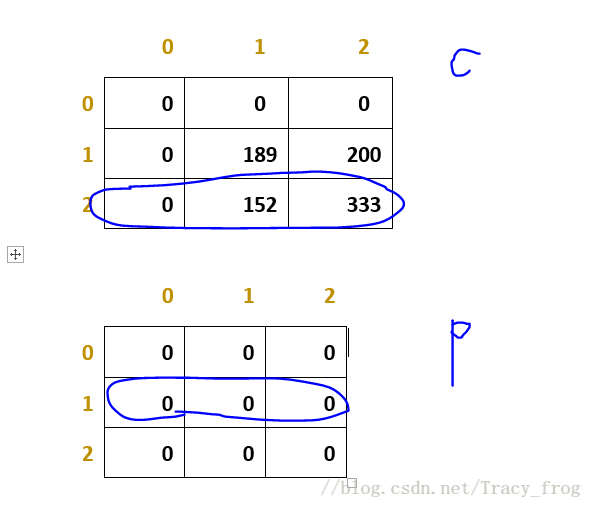c表只用于生成p表，用完就没有作用了，如果我们在生成c表一行的同时生成p表，便只需要一个一维数组c[n+1]存储c表和一个c[2*(n+1)]的二维数组存储p表，空间复杂度下降。 p表能否优化成一维数组呢？ 答案是可以的，因为求p表第i行j列数据时只需要用到c表i行和p表i-1行的前j个数据，我们只需要从后往前遍历，便不会影响到其它数据的计算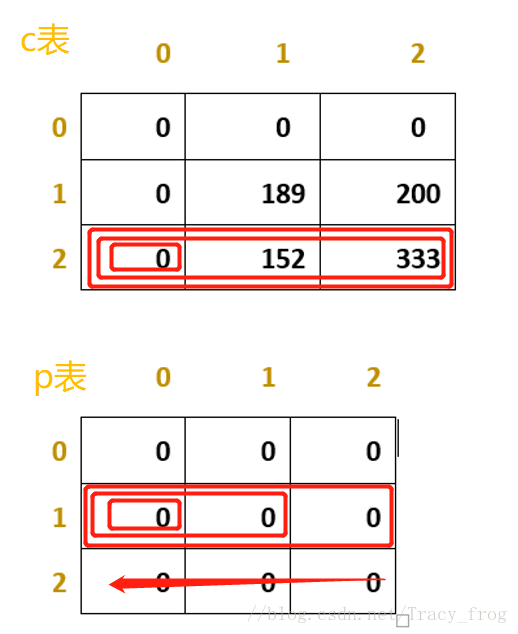优化后代码如下：
void Dynamic_Optimization(int *cc, int *pp)
{
int i, j, k, u;
//最开始最大利润表是0
for(i=0;i<=m;i++)
{
pp[i]=0;
}
cc=0;
for (i = 1; i <= m; i++)//m车间数
{
for (j = 1; j <= n; j++)
{
//初始化一列利润表cc
cc[j] = cc[j - 1] + rand() % 1000 + 1;
}
for (u = n; u >= 1; u--)//从后往前遍历
{
int max = 0;
for (k = u; k >= 0; k--)
{
if (pp[k] + cc[u - k] > max)
{
max = pp[k] + cc[u - k];
pp[u] = max;
}
}
}
}
}

思考：p只是一个一维数组，我们只能通过它得知最大利润，如何得知分配的方案呢？ 如果c和p还是二维数组的时候，其实可以不用分配表application，直接算出分配方案，但现在c和p已经优化为一维数组了，没有application我们无法得知分配方案。 那么application表只能是一个二维数组吗？ 有的博主是这样做的：通过结构体存储，将application表中数值为0的部分省去。结构体内定义了3个int型数据，这种方法比较适合在0较多的application表中用，否则反而可能增加空间负担，得不偿失！请慎用！
结构体定义如下：

Struct path{

Int shebei;

Int chejian;

Int num;

}P;

想到结构体就想到了链表啦，如果像下图这样把每个地方的0压缩，或许可以减少一点空间负担，而且，链表不要求连续的空间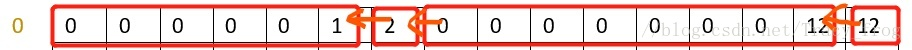如图，把一行变成4个链表块，0的个数保存在链表块里。
展开全文动态规划 优化
• 最优化资源分配问题 问题提出：现有三个发电厂A,B,C其生产成本和最大发电度数分别如下： 发电厂 生产成本T 最大发电度数 A P^2.2 1千万度 B 2p^1.8 1.5千万度 C 0.8p^2.0 1.8千万度 问：全年总发电量不少于3千万度，...
最优化资源分配问题
问题提出：现有三个发电厂A,B,C其生产成本和最大发电度数分别如下： 发电厂 生产成本T 最大发电度数 A P^2.2 1千万度 B 2p^1.8 1.5千万度 C 0.8p^2.0 1.8千万度 问：全年总发电量不少于3千万度，如何分配生产任务才能使公司的总发电成本最低。 问题分析：根据对发电厂发电量的要求，给A,B, C三个发电厂分配不同的发电量，生产成本是不一样的，所以，我们需要寻求的是，既要满足发电要求的发电总量，又要使得发电消耗成本最低。则可以得出优化模型为：Min f=p12.2+2*p21.8+0.8p3^2.0 复合形法： 算法原理：符合形来源于无约束优化问题的单纯形法，通过构造符合形来求最优解，新的复合形通过替换旧的复合形中的坏点（目标函数值最大或次大的点）得到，替换方式仍然是单纯性法中的反射，压缩，扩展这几个基本的方法。 算法步骤：用复合形搜索法求约束优化问题的算法如下： （1）选取复合形{x0,x1,x2,…xn},反射系数 > 1,锁紧系数 （0,1）,扩展系数 > 1,收缩系数 （0,1）及精度 > 0,置k = 0; （2）将复合形的n + 1个顶点按目标函数值的大小 重新编号，f(x0) f(x1) f(x2)…f(xn); （3）令xn+1=1/n∑_(j=0)^(n-1)▒xj,若{1/(n+1) ∑_(j=0)n▒〖[f(xj )-f(x(n+1))]2〗}1/2 ，停止迭代，输出x0,否则转（4）。 （4）计算xn+2=xn+1+ （xn+1-xn）,检查xn+2是否在可行域内，即是否满足g(xn+2) 0，若不在可行域内，将反射系数减小（在后面MATLAB程序中采用开方的形式减小反射系数）直到xn+2在可行域内。计算f(xn+2),若f(xn+2)<f(x0)，转（5）。 （5）计算xn+3=xn+1+ (xn+2-xn+1),检查xn+3是否在可行域内，若不在可行域内，将扩展系数减小（在后面的MATLAB程序中采用开方的形式减小扩展系数），直到xn+3在可行域内。若f(xn+3)<f(x0),令xn=xn+3,转（2），否则，转（6）； （6）令xn=xn+2，转（2）； （7）令xn={xi|f(xi)= min(f(xn),f(xn+2))},计算xn+4=xn+1+ (xn-xn+1)，检查xn+4是否在可行域内，若不在可行域内，将压缩系数减小（在后面的MATLAB程序中采用减半的形式减小压缩系数），直到xn+4在可行域内。 若f(xn+4)<f(xn),令xn=xn+4,转（2），否则转（8）； （8）令xj=x0+ (xj-x0),j=0,1,2…，n,转（2）。
优化模型 目标函数与约束条件分别如下： Min f=p12.2+2*p21.8+0.8*p3^2.0 s.t. p1+p2+p3=3000 0<=p1<=1000 0<=p2<=1500 0<=p3<=1800
代码： Fun.m function f=fun(x) f=x(1)2.2+2*x(2)1.8+0.8*x(3)^2.0;
mycon.m function [c,ceq]=mycon(x) c(1)=-x(1)-x(2)-x(3); ceq=[];
main.m A=[-1 -1 -1]; b=(-3000); vlb=[0;0;0]; vub=[1000;1500;1800]; x0=[0;0;0]; [x,fval]=fmincon(@fun,x0,A,b,[],[],vlb,vub,@mycon);
数据： Fval 2.474853350725768e+06 X [2.852103962403294e+02;1.499999999711306e+03;1.214789604151252e+03]
ABC的 X1=285.21 x2=1500 x3=1214.8 成本 Fval=2474.9
小结： 约束优化的算法基本上可以分为两大类：直接法和间接法。间接法是将约束优化问题转化为无约束优化问题来求解，直接法不需要将问题转化为无约束问题，一般包括可行方法，坐标轮换法等。本次作业探讨的罚函数，比较关键的是要找好罚函数。在复合形法中，需要给出初始的复合形，而初始复合形必须在可行域内，初始复合形可人工给出，也可利用随机法产生，根据难易程度自行决定，但为了满足可行域条件，必须检查每个顶点是否在可行域内，这是很关键的一步。
展开全文最优化 MATLAB
• Lingo与一维资源分配问题 代码如下： sets: R/1..6/:z; L/1..3/; c(R,L):x,y; endsets data: X=0 0 0 5 5 4 15 15 26 40 40 40 80 60 45 90 70 50; z=0 1 2 3 4 5; enddata max=@sum(c(i,j):X(i,j)*y(i...
Lingo与一维资源分配问题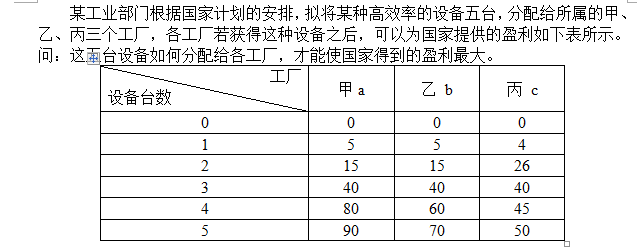代码如下：
sets:
R/1..6/:z;
L/1..3/;
c(R,L):x,y;
endsets
data:
X=0 0 0
5 5 4
15 15 26
40 40 40
80 60 45
90 70 50;
z=0 1 2 3 4 5;
enddata
max=@sum(c(i,j):X(i,j)*y(i,j));
@for(l(i):
@sum(c(j,k)|k#eq# 1:y(j,k))=1);
@sum(c(i,j):y(i,j)*z(i))=5;
@for(c(i,j):@B in(y(i,j)));
end

展开全文• 想要明白调度过程中的资源分配问题，首先要明白一件事，k8s中的资源分配，是以Pod为单位的。而Pod本质上而言，其实就是一个进程。就像操作系统一样，进程是资源分配的最小单位。 k8s解决的一个比较大的问题，就是...
• 资源分配问题是将数量一定的一种或若干种资源(原木料、资金、设备或劳动力等)合理地分配给若干个使用者,使总收益最大。 例如,某公司有3个商店A、B、C，拟将新招聘的5名员工分配给这3个商店，各商店得到新员工后每年...动态规划 算法
• 资源分配问题是考虑如何把有限分配给若干个工程的问题。参考《算法设计与分析》 下面直接贴代码： //为了和书上的内容一致，我的变量名、变量所代表的意思和书上的几本一致 #include #include #define M 8 //可...
• 资源分配问题是将数量一定的一种或若干种资源（原材料、资金、设备或劳动力等），合理地分配给若干使用者，使总收益最大。 例如，某公司有3个商店A、B、C，拟将新招聘的5名员工分配给这3个商店，各商店得到新员工后...动态规划 算法
• 论文研究- 具有合作关系的多人递阶资源分配问题研究.pdf, 资源分配问题是一个复杂的管理问题,它所解决的是上级如何将有限的资源在所属部门之间进行分配,而使它自己的与...
• 实验名称：用动态规划法求解资源分配问题 （验证型实验） 实验目标： （1）掌握用动态规划方法求解实际问题的基本思路。 （2）进一步理解动态规划方法的实质，巩固设计动态规划算法的基本步骤。 实验任务： （1）...
• 问题举例二：资源分配问题 例 5.1.2 离散变量的资源分配问题 多阶段决策问题 动态规划的最优子结构性质 动态规划的子问题重叠性质 前向优化 后向优化 例 5.1.2 连续变量的资源分配问题 例 5.3.2 多阶段有限资源分配...动态规划 数学建模 最短路问题
• 常常发生队列得不到资源的情况，即使没有达到fairshare阈值。 基础知识准备： 首先，需要了解fairscheduler的调度原则是什么样的： ... 队列得不到资源分配问题 > image2017-1-5 21:15:15.png" class
• 资源分配问题：某厂根据计划安排，拟将n台相同的设备分配给m个车间，各车间获得这种设备后，可以为国家提供盈利Ci j(i台设备提供给j号车间将得到的利润，1≤i≤n，1≤j≤m)。问如何分配，才使国家得到最大的盈利？ ...动态规划
• 某厂根据计划安排，拟将n台相同的设备分配给m个车间，各车间获得这种设备后，可以为国家提供盈利Ci j(i台设备提供给j号车间将得到的利润，1≤i≤n，1≤j≤m) 。问如何分配，才使国家得到最大的盈利？
• 有关资源分配问题。数学建模的格式什么的可以借鉴一下。我们自己写的。题目比较简单。适合初学者学习一下。
• 资源分配问题: 是考虑如何把有限的资源分配给 若干个工程问题。假设资源总数为 r，工程个数为 n，给每个工程投入的资源不同，所得的利润也不 同，要求把总数为 r 的资源分配给n个工程，以获 得最大利润的分配方案。 ...算法设计与分析 动态规划
• 分配超出 worker 可用的内存容量，大家根据自己 worker 的容量进行分配任务资源。 如果使用配置—executor-cores,超过了每个 worker 可以的 cores，任务处于等待状态。 如果使用—total-executor-cores ,即使...
• 在银行家算法中，若出现表2-4所示的资源分配情况，试问： 该状态是否安全？ 如果进程P2提出请求Request2(1,2,2,2)后，系统能否将资源分配给它。 表2-4 资源分配表 Allocation Need ...操作系统
• 实验名称：用动态规划法求解资源分配问题 （验证型实验） 实验目标： (1)掌握用动态规划方法求解实际问题的基本思路。 (2)进一步理解动态规划方法的实质，巩固设计动态规划算法的基本步骤。 实验任务： (1)...算法设计
• 问题描述：给出一定用户的请求（各类虚拟机的数量），装在给定规格的服务器内 ，要求服务器的资源利用率最大。其中，虚拟机和服务器各有两个维度限制，CPU和内存（Mem）。思路：本题实质是背包问题，考虑过dp，贪心...智能算法 优化算法
• 实验目标： （1）掌握用动态规划方法求解实际问题的基本思路。...（1）设计动态规划算法求解资源分配问题，给出算法的非形式描述。  （2） 在Windows环境下用C 语言实现该算法。计算10个实例，每个实例中n=30，
• #include<iostream>...//资源最大利润分配表 float q,f,temp;//利润表 当前最大收益 正在计算的最大收益 printf("输入工程数m:"); scanf("%d",&m); printf("输入总资源数n:...算法
• 一维资源分配问题 二维资源分配问题 固定资金分配问题 转载于:...
• 问题描述】 某公司有3个商店A, B, C，拟将新招聘的5名员工分配给这3个商店，各商店得到的新员工后每年的赢利情况如表所示，求如何分配才能使利益最大化？ 表 （右边数字是每个公司分配0 – 5个人的收益情况） ...算法...